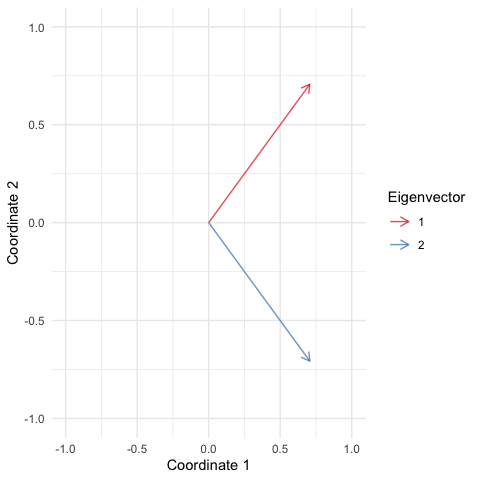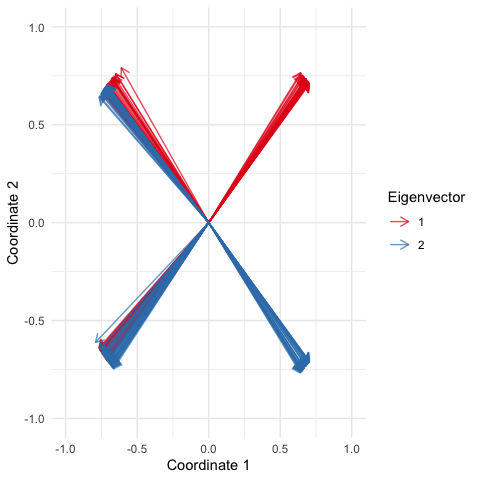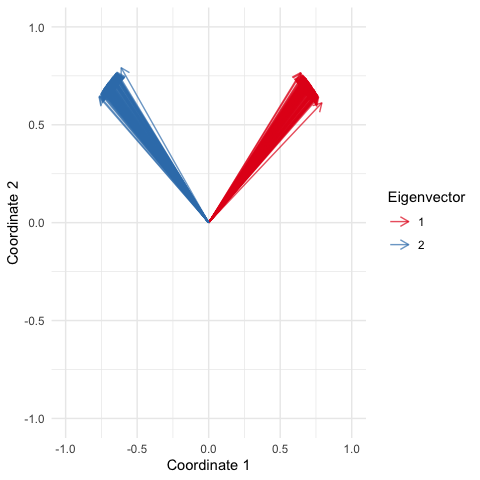# Introduction to RcppHungarian

The RcppHungarian package solves one problem well. Namely, it solves the minimum cost bipartite matching problem. Here is an example of the problem:

Suppose you have $$N_H$$ humans and $$N_D$$ dogs that need adoption. Each human wants a dog and each dog wants a human. However humans and dogs may differ in their energy levels. A high-energy dog can ruin a house if he doesn’t get enough exercize and a high-energy human may be annoyed if their dog is not up for their desired activities. Therefore you could imagine there is some “cost” of assigning dog $$j$$ to human $$i$$ we we could denote $$C_{ij}$$. The problem is to find the optimal matching between dogs and humans to minimize cost. Finally we require that whichever group has fewer (fewer dogs or fewer humans) must be fully matched while the larger group will have some people/dogs that don’t get matched.

In other words the problem can be formalized as: given an $$N_H$$ by $$N_D$$ cost matrix $$C$$ find the optimal set of $$m=\text{min}\{N_H, N_D\}$$ matchings $$(i, j)$$ where $$i\in \{1,\dots,N_H\}$$ and $$j\in \{1,\dots,N_D\}$$ such that the total cost of the matchings $$\sum_m C_{ij}$$ is minimized.

The RcppHungarian package solves this type of problem (given $$C$$) using something called the Hugarian algorithm (aka the Huhn-Munkres algorithm). This package wraps the C++ code written by Cong Ma in the hungarian-algorithm-cpp library on GitHub. I have wrapped/edited that code in such a way that the algorithm can be used either through the R interface HungarianSolver or as a header-only C++ library which can be included in other packages.

# A Simple Example

To give an example usage lets solve that dog problem supposing the following cost matrix

set.seed(593903)

library(RcppHungarian)
library(ggplot2)

# humans are rows (5), dogs are columns (4)
cost <- rbind(c(1,5,2,19),
c(4,0,3,10),
c(6,9,6,1),
c(9,8,1,3),
c(6,1,1,1))
HungarianSolver(cost)
#> $cost #>  3 #> #>$pairs
#>      [,1] [,2]
#> [1,]    1    1
#> [2,]    2    2
#> [3,]    3    4
#> [4,]    4    3
#> [5,]    5    0

So the algoritym has run and we see that the overall minimum cost is 3. Additionally we now know that person 1 should adopt dog 1, person 2 dog 2, persion 3 dog 4, and person 4 dog 3. Sadly the algoritym also tells us that there is no dog for person 5 that minimizes overall cost.

# A More Complicated Example

I will admit, the above example is pretty contrived. Lets consider a more complicated example that was my acctual motivation for creating this package. Lets say we have a posterior distribution over covariance matricies and we want to visualize the posterior distribution of the first and second eigenvectors of these matrices. Importantly, we care about visualizing the “principle directions of variation” not just what the algorithm tells us are the first and second eigenvectors (this distnction should be clear in a second).

I start by simulating a distributio over covariance matricies. Here I am going to do it in a bit of a contrived way to show my point. I am going to simulate covariace matricies from a set of fixed eigenvectors and just two eigenvalues one that is bigger than the other. Yet I am going to randomly select which of the two eigenvectors gets the larger eigenvalue. In other words I have a bunch of covariance matricies which have the same rotation, but different scaling" along that rotation. Finally I add a bit of Wishart noise to ensure that the eigenvectors are not exactly the same just very very close to the true values - this just makes the plots prettier.

# Create a distribution of matricies that have identical eigenvectors but there
# is randomness in the eigenvalues and a tiny bit of added noise from a wishart
const <- 1/sqrt(2)
V <- cbind(c(const,const), c(const,-const))
Sigma <- array(0, dim=c(2, 2, 100))
for (i in 1:dim(Sigma)){
Sigma[,,i] <- V %*% diag(sample(c(1, 1.3))) %*% t(V) + rWishart(1, 50, 0.002*diag(2))[,,1]
}

Just as a reference here is a plot of the true eigenvectors shared by all matricies in the sample

plot_arrows(V, c("1", "2"))We could take the eigen decomposition of each covariance matrix and then just plot the distribution over the first and second eigenvectors but there is are two problems:

1. The ordering of the eigenvector is defined by the ranking of the eigenvalues.
2. Transform is only defined up to the sign of the eignevectors.

The first problem implies that the “direction” reflected in the first eigenvalue of one sample might acctually correspond to the second eigenvalue in the second sample etc… Therefore we will need some method of matching “directions” between samples (get it?… we are going to do this matching with the Hungarian algoritym)

The second problem implies that we will have to be smart about how we do that matching, we acctually might need to match an eigenvector $$v$$ in one sample with the eigenvector $$-u$$ in a second (i.e., we may need to match to the negative of vector).

So lets do it the naive way and see what happens.

# Decompose each sample
Sigma.vectors <- array(0, dim=dim(Sigma))
for (i in 1:dim(Sigma)){
decomp <- eigen(Sigma[,,i])
Sigma.vectors[,,i] <- decomp$vectors } # Plot distribution of the first two Eigenvectors dat <- rbind(t(Sigma.vectors[,1,]), t(Sigma.vectors[,2,])) col <- c(rep("1", dim(Sigma)), rep("2", dim(Sigma))) plot_arrows(dat, col)Hard to interpret right? Weirdly there are eigenvectors going each of 4 directions for some reason with their lables all mixed up and some +/- weirdness happening. So as I alluded to before, we will solve this using the Hungarian algoritym. We are going to pick a reference sample (in this case the first sample) and match the eigenvectors of each sample against the eigenvectors of the reference. In this case our “cost matrix” will be the pairwise euclidean distance between eigenvectors from the reference and eigenvectors of the sample to be matched. Notably, to deal with the sign issues (problem 2 above) we will use a trick, every eigenvector in the sample will be doubled (the second copy is the negation of the first). The following code does all this.  # Here is our distance function - calculates the pairwise distance # between two sets of vectors. cost_distance <- function(V1, V2){ n1 <- ncol(V1) n2 <- ncol(V2) D <- as.matrix(dist(t(cbind(V1, V2)))) return(D[1:n1, (n1+1):(n1+n2)]) } # Make some empty containers to store results Sigma.vectors <- array(0, dim=dim(Sigma)) # Initialize n=1 case - this is our reference against which # we will match the rest of the samples decomp <- eigen(Sigma[,,1]) Sigma.vectors[,,1] <- decomp$vectors

# Now match up each sample with the reference
for (i in 2:dim(Sigma)){
decomp <- eigen(Sigma[,,i])
Sigma.vectors[,,i] <- decomp$vectors # Here we add a second (negated) copy of the eigenvectors in the sample Sigma.vectors.expanded <- cbind(Sigma.vectors[,,i], -Sigma.vectors[,,i]) # Here we compute the "cost matrix" (note this is rectangular) # because we have the duplicated (negated) vectors from the sample cost <- cost_distance(Sigma.vectors[,,1],Sigma.vectors.expanded) # This is the key line where we solve the matching problem matching <- RcppHungarian::HungarianSolver(cost) # This is the key line were we reorder the eigenvectors / select if we wanted # the negative or positive versions Sigma.vectors[,,i] <- Sigma.vectors.expanded[,matching$pairs[,2]]
}

# Now we check our work visually
dat <- rbind(t(Sigma.vectors[,1,]),
t(Sigma.vectors[,2,]))
col <- c(rep("1", dim(Sigma)), rep("2", dim(Sigma)))
plot_arrows(dat, col)Looks much better right? I woudl say this is about as good as this is going to get. Note, it may still not look identical to the original (the true eigenvectors) because we don’t know the true sign of the eigenvectors but the overall information conveyed by our result is identical to the original.

# Custom Functions

If you want to see what was in that plot_arrows function:

plot_arrows
#> function(dat, col){
#>   dat <- data.frame(dat, group=col)
#>   colnames(dat) <- c("Coordinate 1", "Coordinate 2", "Eigenvector")
#>   ggplot(dat) +
#>     geom_segment(aes(xend=Coordinate 1, yend=Coordinate 2, x=0, y=0, color=Eigenvector),
#>                  arrow=arrow(length=unit(0.1, "inches")), alpha=0.7) +
#>     theme_minimal()+
#>     xlab("Coordinate 1") +
#>     ylab("Coordinate 2")+
#>     scale_color_brewer(palette="Set1") +
#>     ylim(c(-1,1))+
#>     xlim(c(-1,1))
#> }
#> <bytecode: 0x7fee42f34240>